Скачать презентацию Algorithms wait Math Everywhere Gerald Kruse Ph

506550b2d313cd0722bf5beaaa79bd0b.ppt

• Количество слайдов: 58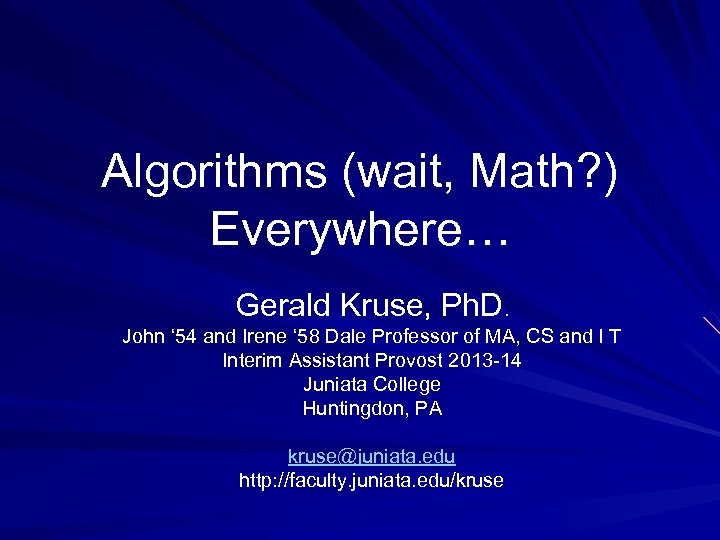Algorithms (wait, Math? ) Everywhere… Gerald Kruse, Ph. D. John ‘ 54 and Irene ‘ 58 Dale Professor of MA, CS and I T Interim Assistant Provost 2013 -14 Juniata College Huntingdon, PA [email protected] edu http: //faculty. juniata. edu/kruseSome Context / Confessions… Prepare to be underwhelmed. I can’t return the hour or so you spend here. I am impressed by the elegance of the algorithms I will present today, and I will probably try too hard to explain the underlying math (“but it’s so cool…”). We like and depend on many automated processes, we just have issues implementing or interacting with them. But, when we understand an algorithm, we can manipulate it. (my CS 315 students “Google Bombed” Juniata… in a good way…). Are we really surprised to learn that a Google search isn’t “free? ”What movie should we pick? \$1, 000 to the first algorithm that was 10% better than Netflix’s original algorithm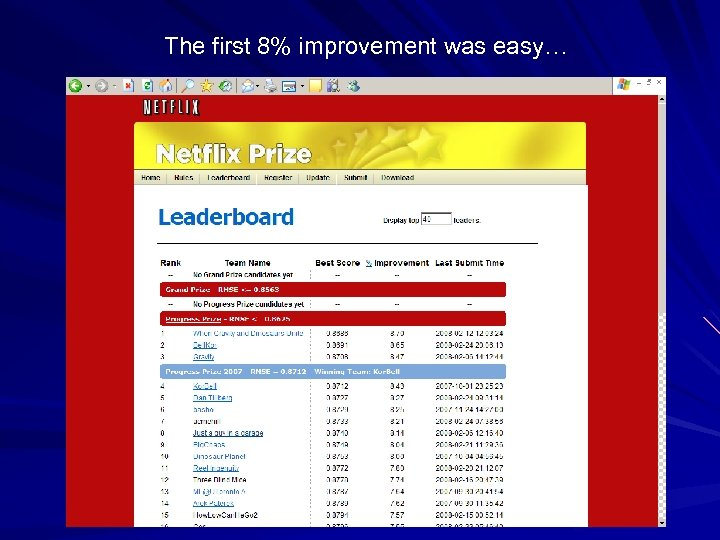The first 8% improvement was easy…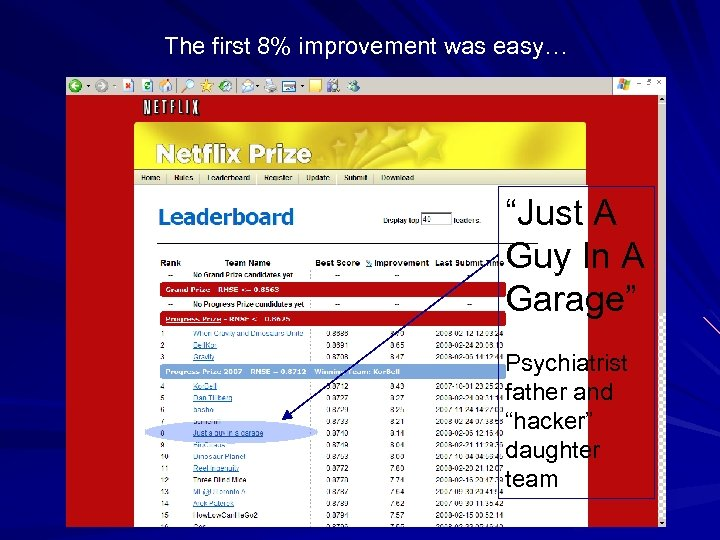The first 8% improvement was easy… “Just A Guy In A Garage” Psychiatrist father and “hacker” daughter team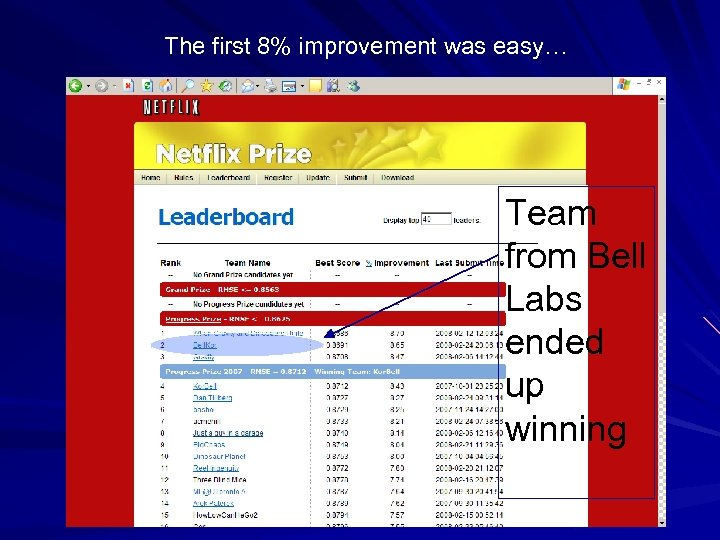The first 8% improvement was easy… Team from Bell Labs ended up winningHere’s an interesting billboard, from a few years ago in Silicon Valley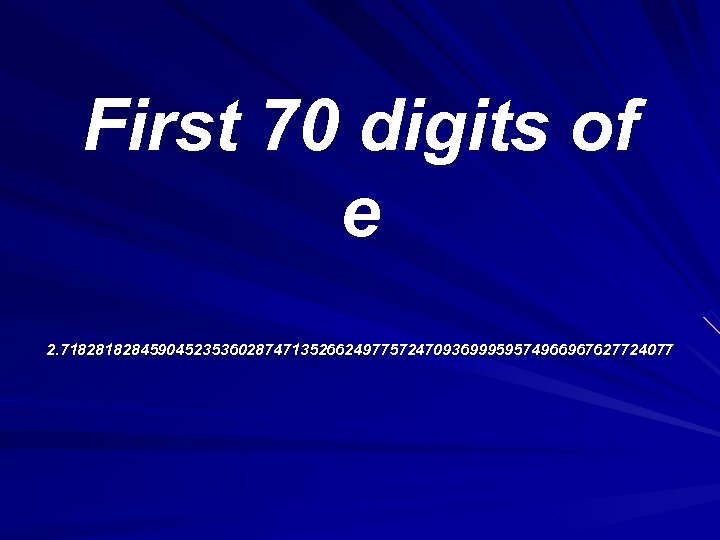First 70 digits of e 2. 71828459045235360287471352662497757247093699959574966967627724077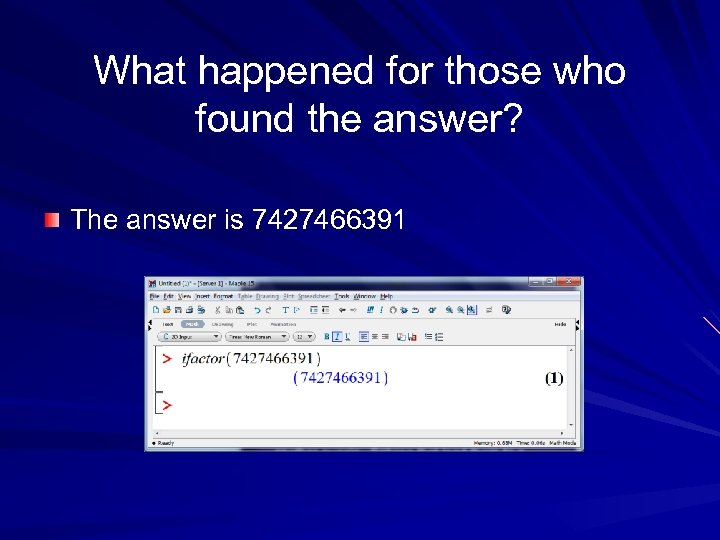What happened for those who found the answer? The answer is 7427466391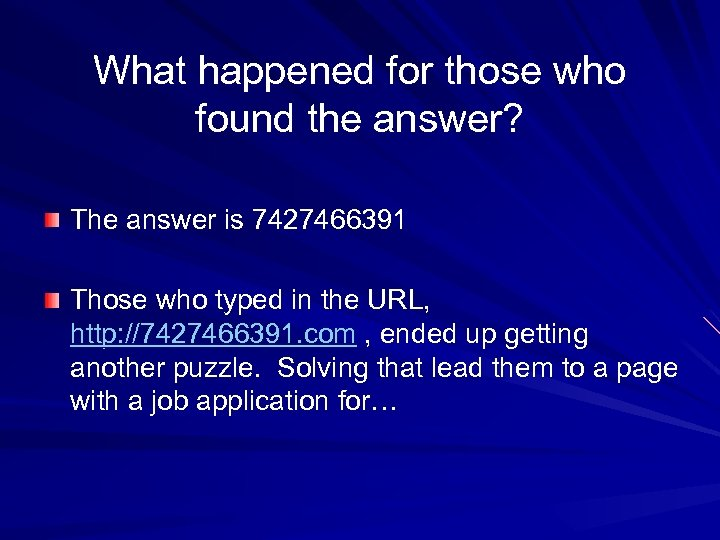What happened for those who found the answer? The answer is 7427466391 Those who typed in the URL, http: //7427466391. com , ended up getting another puzzle. Solving that lead them to a page with a job application for…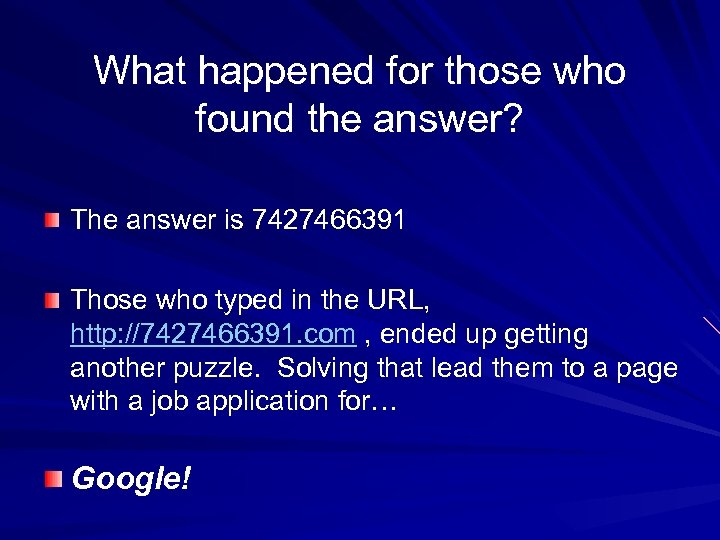What happened for those who found the answer? The answer is 7427466391 Those who typed in the URL, http: //7427466391. com , ended up getting another puzzle. Solving that lead them to a page with a job application for… Google!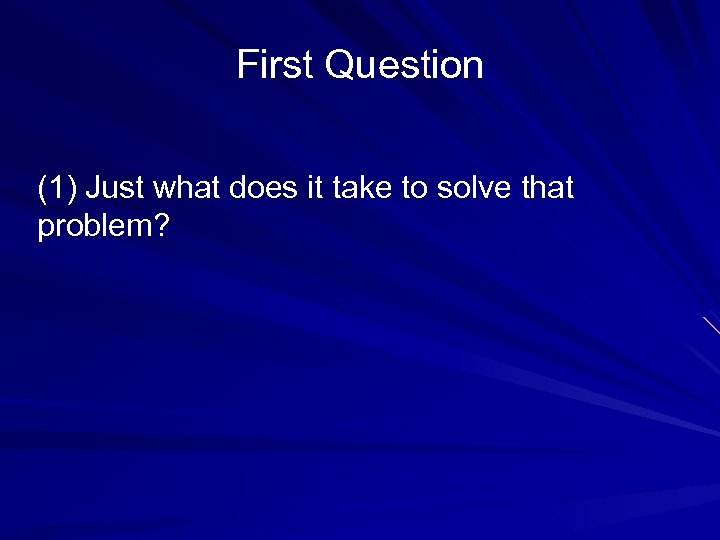First Question (1) Just what does it take to solve that problem?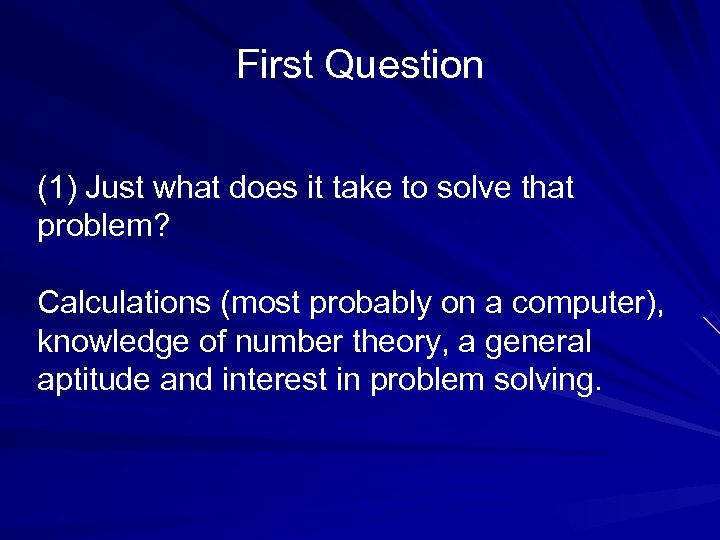First Question (1) Just what does it take to solve that problem? Calculations (most probably on a computer), knowledge of number theory, a general aptitude and interest in problem solving.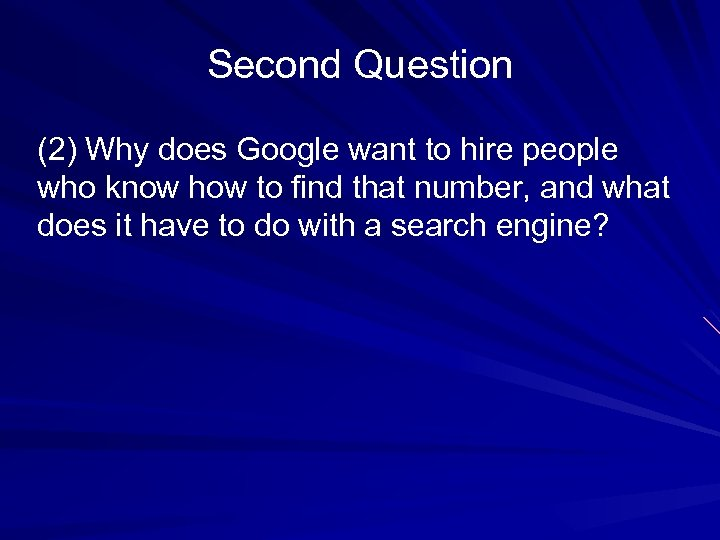Second Question (2) Why does Google want to hire people who know how to find that number, and what does it have to do with a search engine?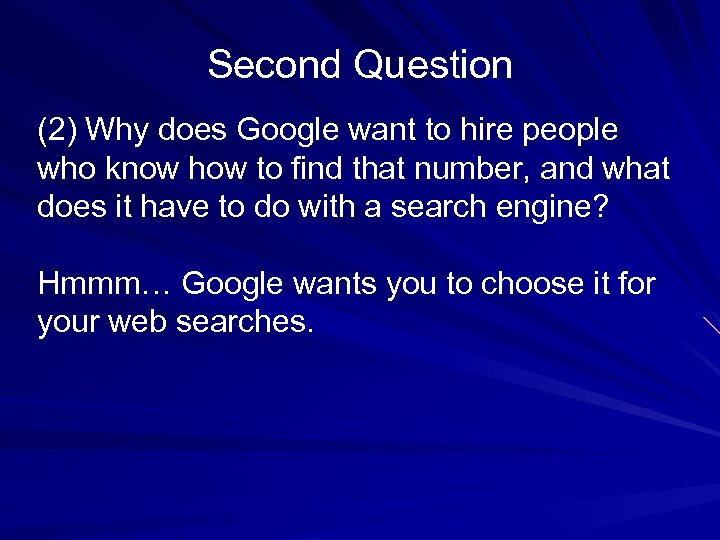Second Question (2) Why does Google want to hire people who know how to find that number, and what does it have to do with a search engine? Hmmm… Google wants you to choose it for your web searches.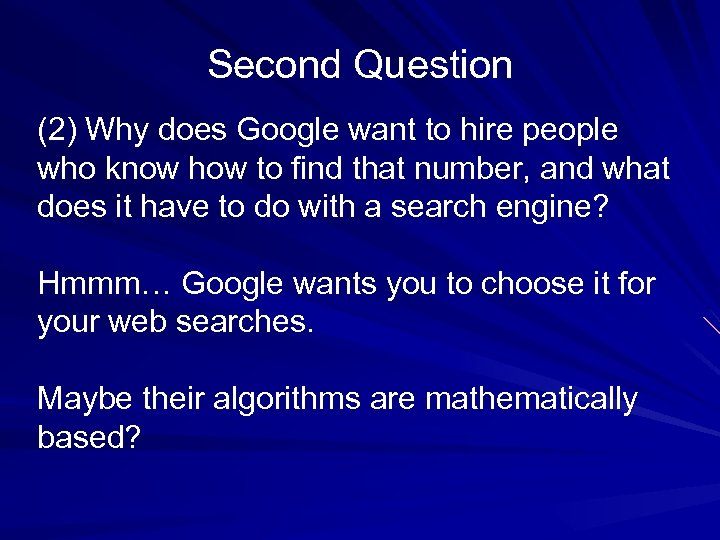Second Question (2) Why does Google want to hire people who know how to find that number, and what does it have to do with a search engine? Hmmm… Google wants you to choose it for your web searches. Maybe their algorithms are mathematically based?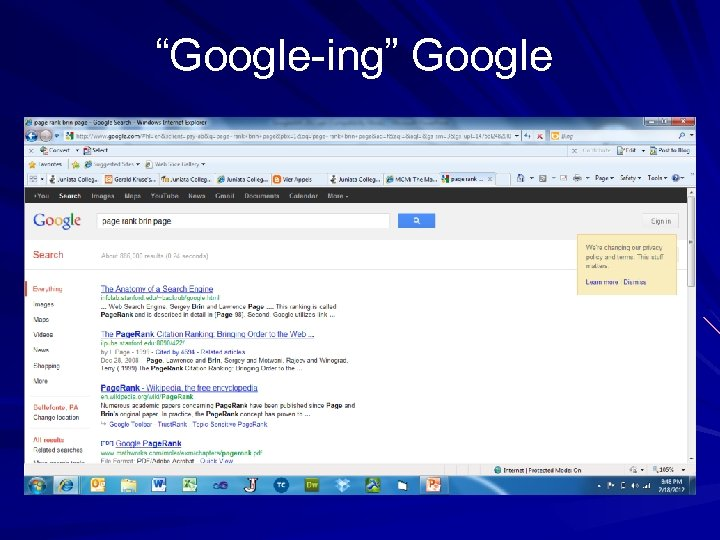“Google-ing” Google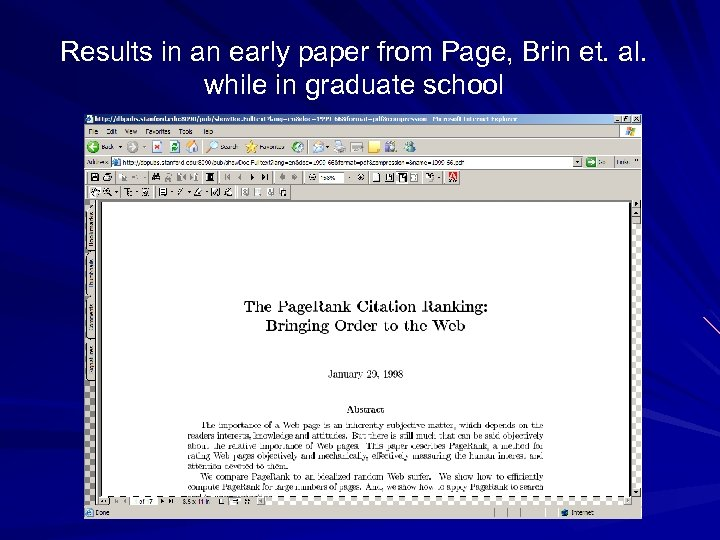Results in an early paper from Page, Brin et. al. while in graduate school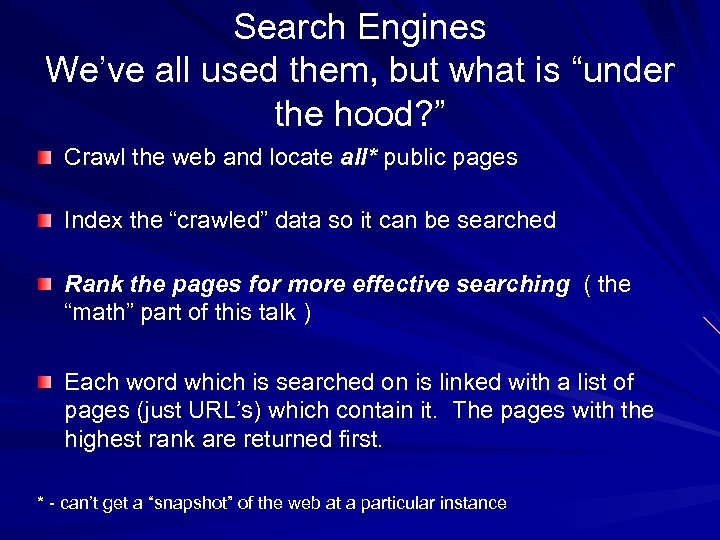Search Engines We’ve all used them, but what is “under the hood? ” Crawl the web and locate all* public pages Index the “crawled” data so it can be searched Rank the pages for more effective searching ( the “math” part of this talk ) Each word which is searched on is linked with a list of pages (just URL’s) which contain it. The pages with the highest rank are returned first. * - can’t get a “snapshot” of the web at a particular instance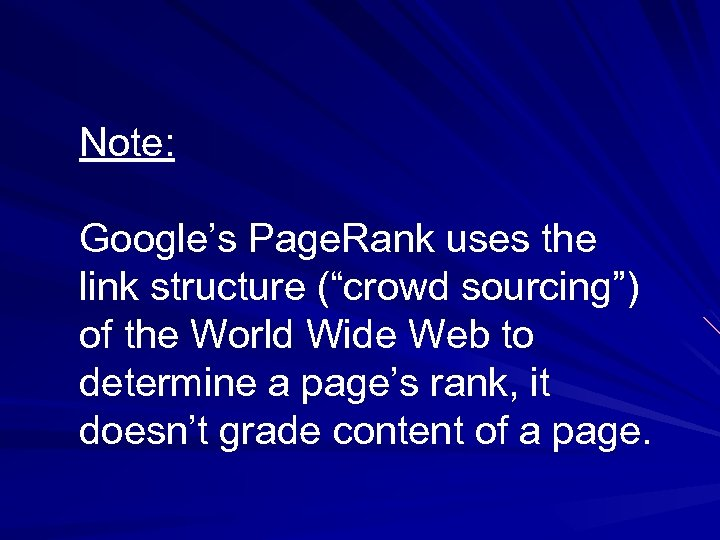Note: Google’s Page. Rank uses the link structure (“crowd sourcing”) of the World Wide Web to determine a page’s rank, it doesn’t grade content of a page.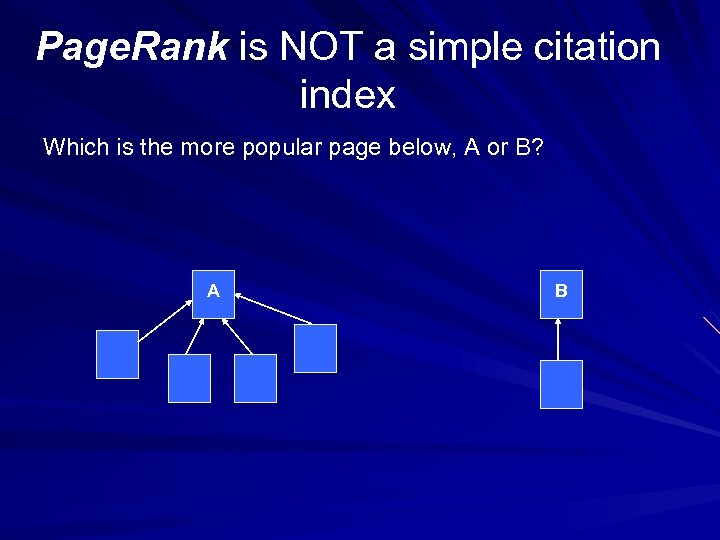Page. Rank is NOT a simple citation index Which is the more popular page below, A or B? A B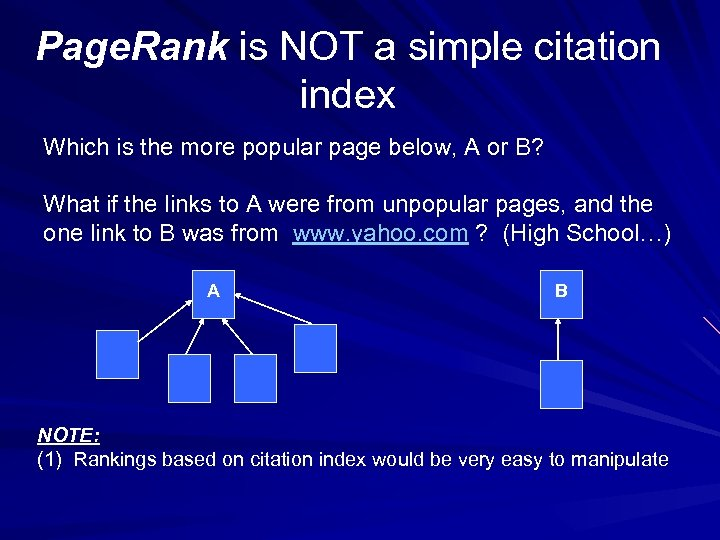Page. Rank is NOT a simple citation index Which is the more popular page below, A or B? What if the links to A were from unpopular pages, and the one link to B was from www. yahoo. com ? (High School…) A B NOTE: (1) Rankings based on citation index would be very easy to manipulate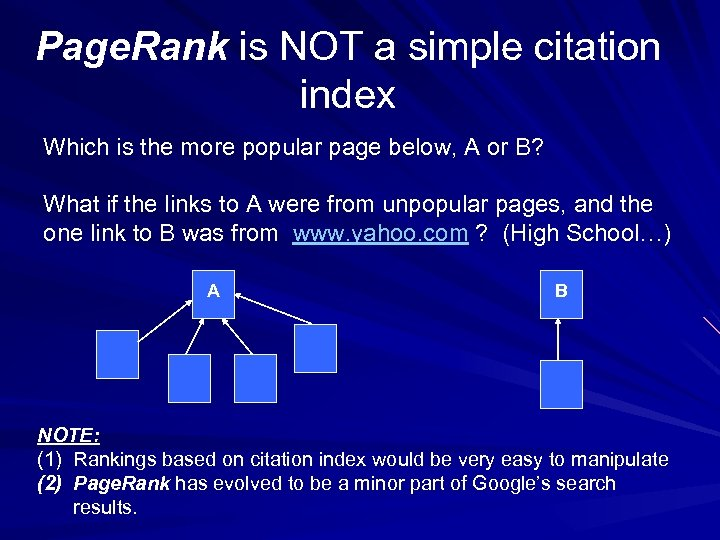Page. Rank is NOT a simple citation index Which is the more popular page below, A or B? What if the links to A were from unpopular pages, and the one link to B was from www. yahoo. com ? (High School…) A B NOTE: (1) Rankings based on citation index would be very easy to manipulate (2) Page. Rank has evolved to be a minor part of Google’s search results.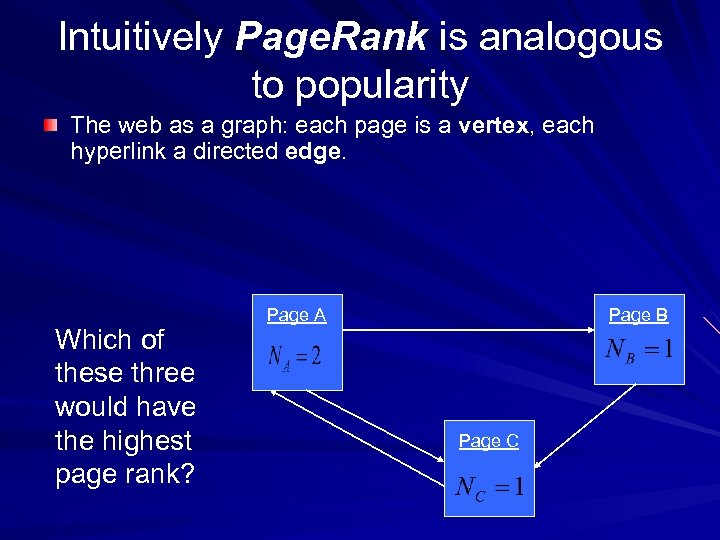Intuitively Page. Rank is analogous to popularity The web as a graph: each page is a vertex, each hyperlink a directed edge. Which of these three would have the highest page rank? Page A Page B Page C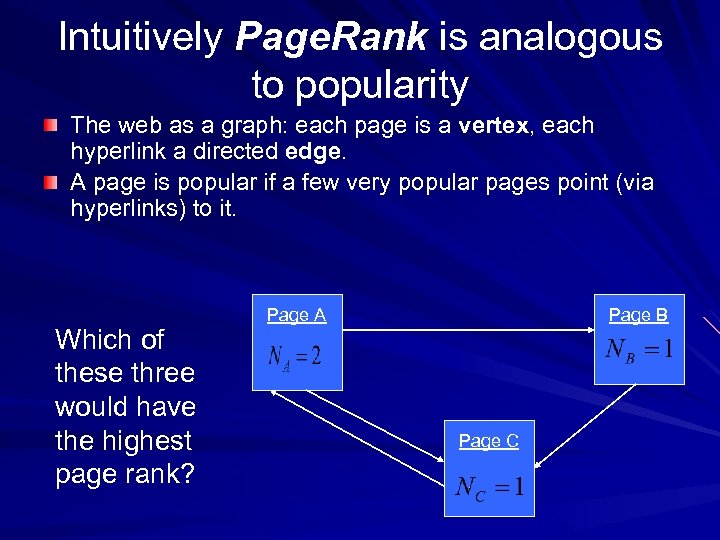Intuitively Page. Rank is analogous to popularity The web as a graph: each page is a vertex, each hyperlink a directed edge. A page is popular if a few very popular pages point (via hyperlinks) to it. Which of these three would have the highest page rank? Page A Page B Page C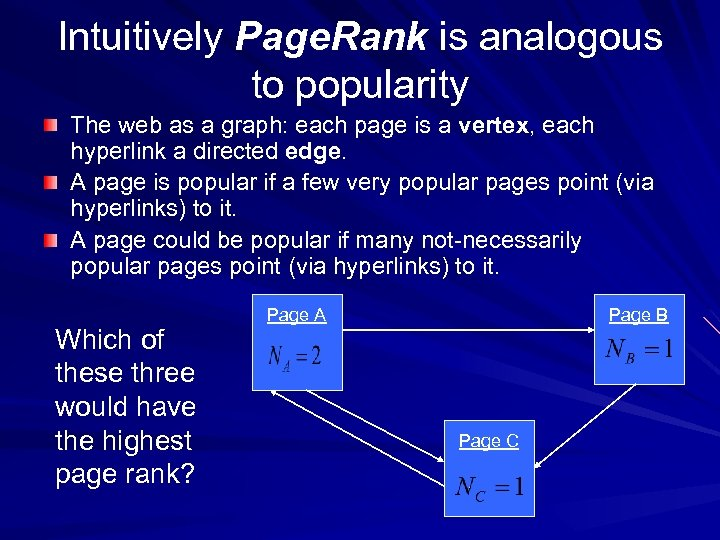Intuitively Page. Rank is analogous to popularity The web as a graph: each page is a vertex, each hyperlink a directed edge. A page is popular if a few very popular pages point (via hyperlinks) to it. A page could be popular if many not-necessarily popular pages point (via hyperlinks) to it. Which of these three would have the highest page rank? Page A Page B Page C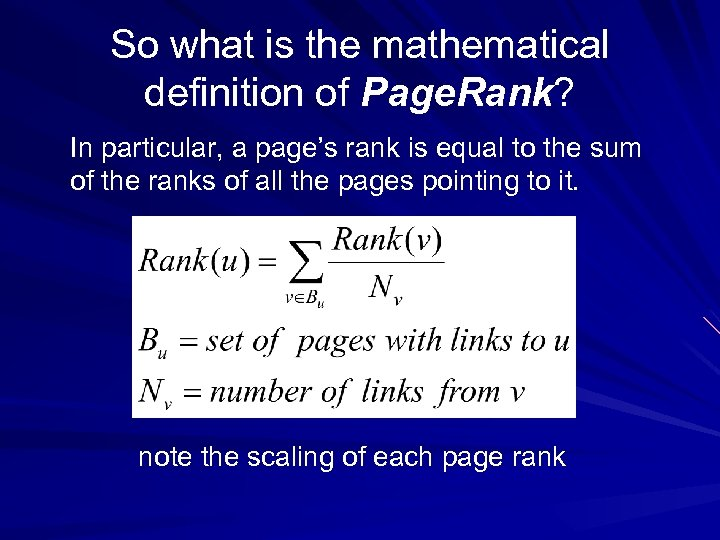So what is the mathematical definition of Page. Rank? In particular, a page’s rank is equal to the sum of the ranks of all the pages pointing to it. note the scaling of each page rank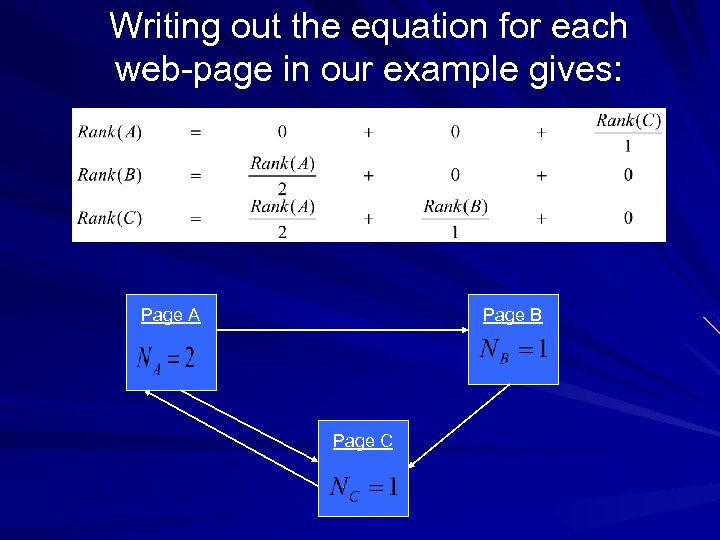Writing out the equation for each web-page in our example gives: Page A Page B Page C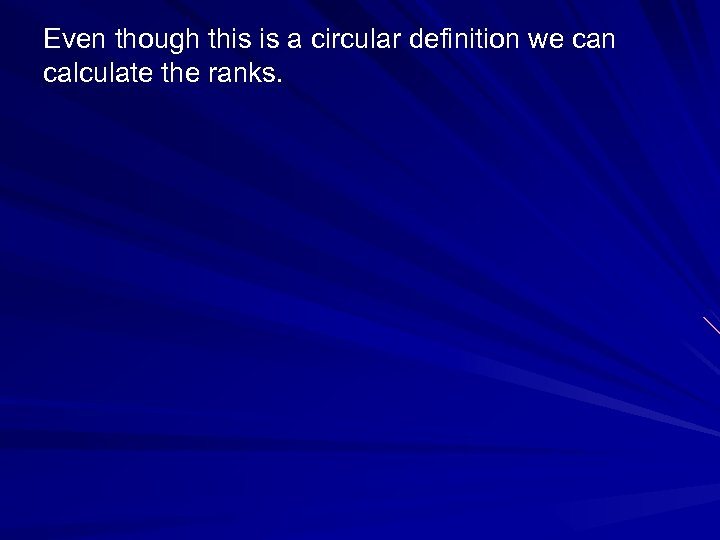Even though this is a circular definition we can calculate the ranks.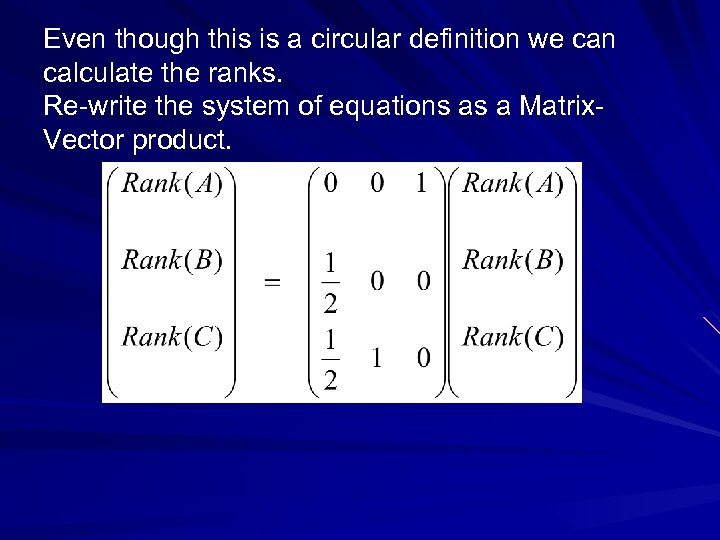Even though this is a circular definition we can calculate the ranks. Re-write the system of equations as a Matrix. Vector product.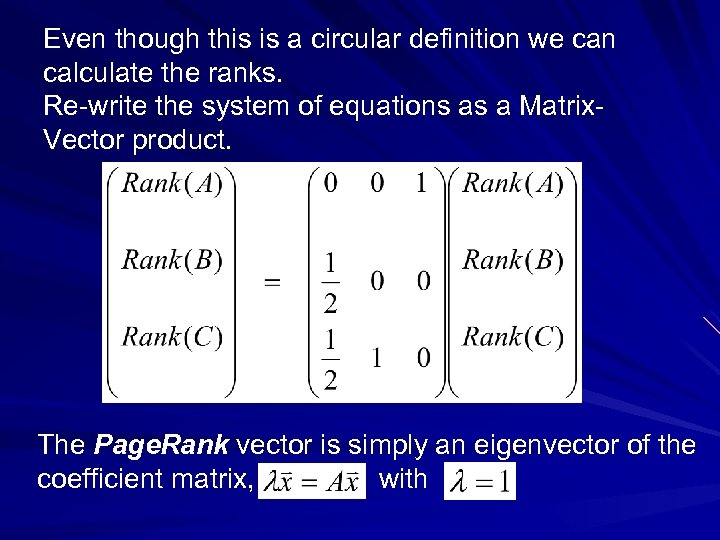Even though this is a circular definition we can calculate the ranks. Re-write the system of equations as a Matrix. Vector product. The Page. Rank vector is simply an eigenvector of the coefficient matrix, withWait… what’s an eigenvector?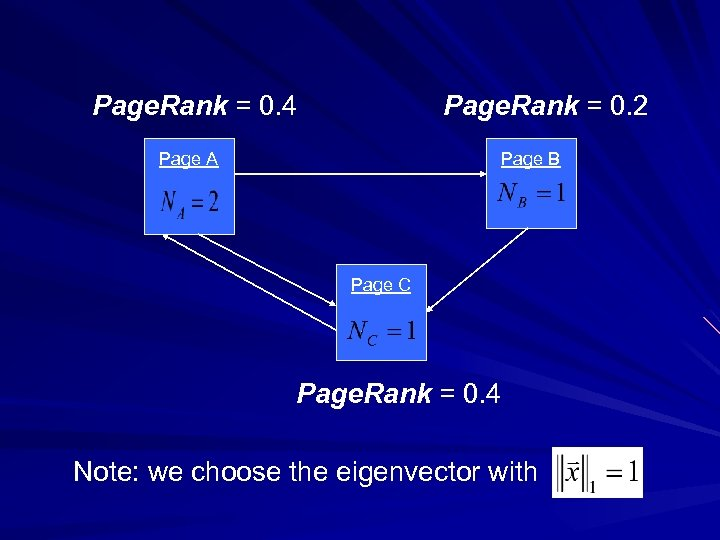Page. Rank = 0. 4 Page. Rank = 0. 2 Page A Page B Page C Page. Rank = 0. 4 Note: we choose the eigenvector with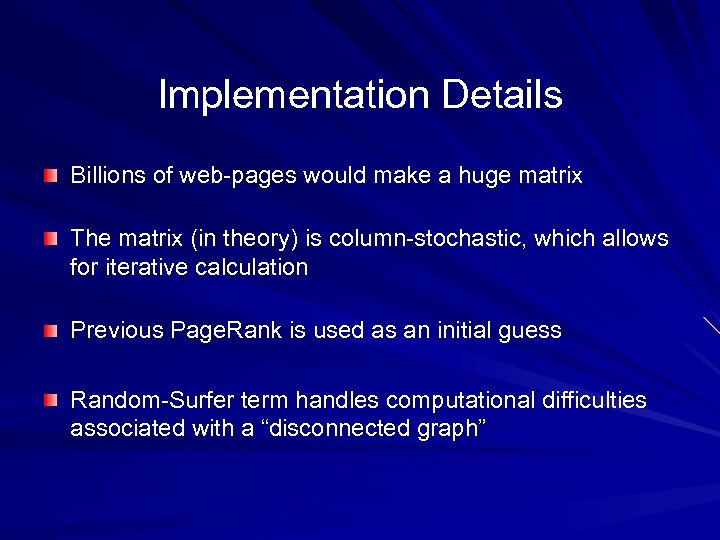Implementation Details Billions of web-pages would make a huge matrix The matrix (in theory) is column-stochastic, which allows for iterative calculation Previous Page. Rank is used as an initial guess Random-Surfer term handles computational difficulties associated with a “disconnected graph”Wait… what else gets searched?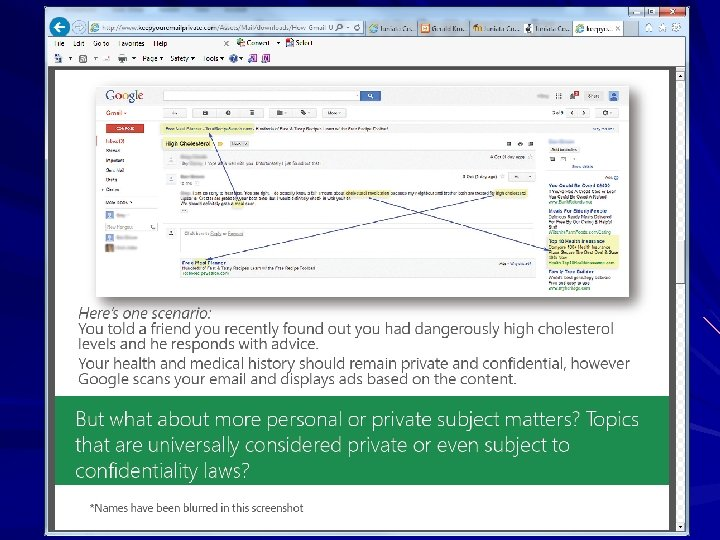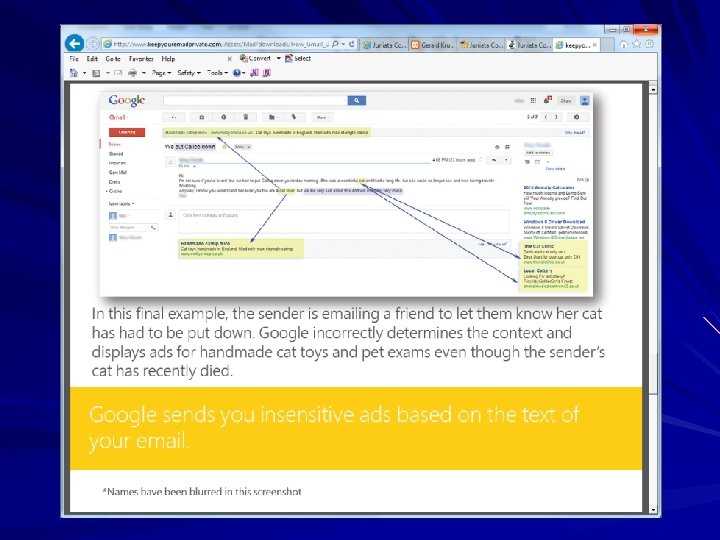Attempts to Manipulate Search Results Via a “Google Bomb”Liberals vs. Conservatives! In 2007, Google addressed Google Bombs, too many people thought the results were intentional and not merely a function of the structure of the webJuniata’s own “Google Bomb”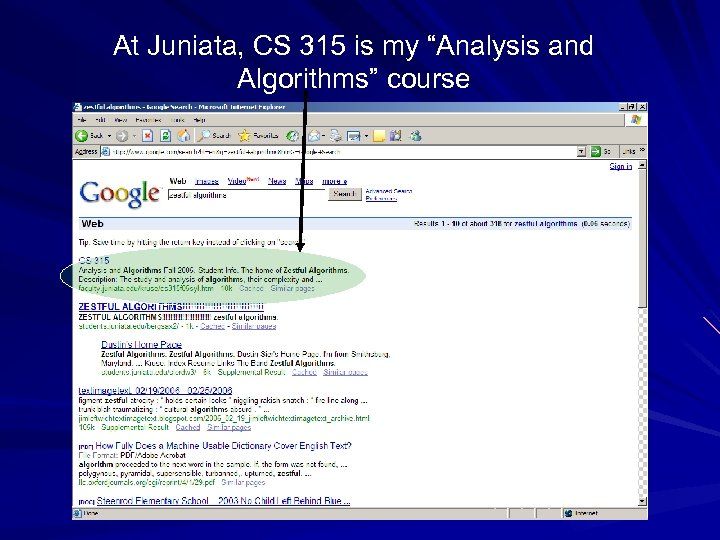At Juniata, CS 315 is my “Analysis and Algorithms” course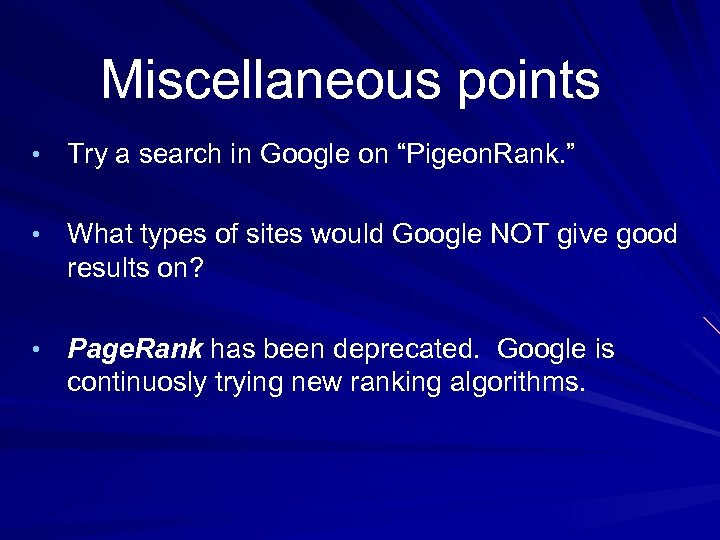Miscellaneous points • Try a search in Google on “Pigeon. Rank. ” • What types of sites would Google NOT give good results on? • Page. Rank has been deprecated. Google is continuosly trying new ranking algorithms.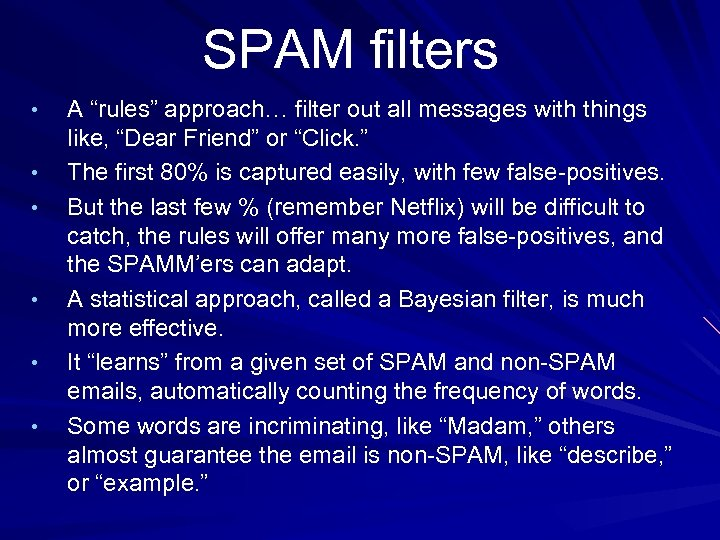SPAM filters • • • A “rules” approach… filter out all messages with things like, “Dear Friend” or “Click. ” The first 80% is captured easily, with few false-positives. But the last few % (remember Netflix) will be difficult to catch, the rules will offer many more false-positives, and the SPAMM’ers can adapt. A statistical approach, called a Bayesian filter, is much more effective. It “learns” from a given set of SPAM and non-SPAM emails, automatically counting the frequency of words. Some words are incriminating, like “Madam, ” others almost guarantee the email is non-SPAM, like “describe, ” or “example. ”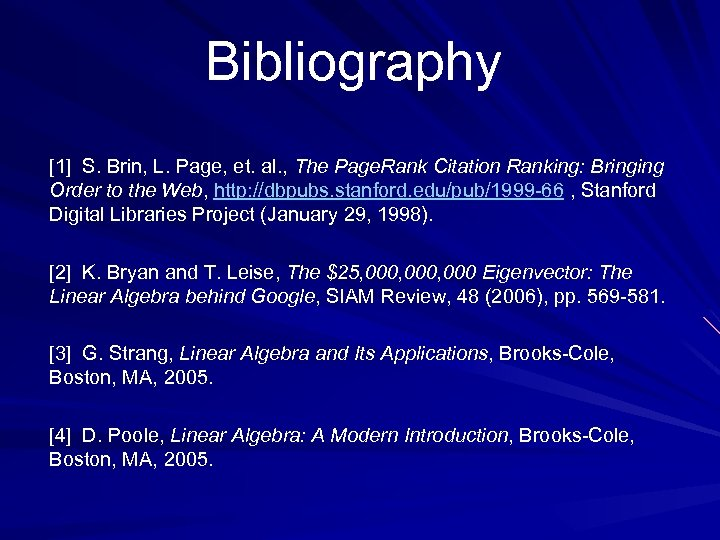Bibliography  S. Brin, L. Page, et. al. , The Page. Rank Citation Ranking: Bringing Order to the Web, http: //dbpubs. stanford. edu/pub/1999 -66 , Stanford Digital Libraries Project (January 29, 1998).  K. Bryan and T. Leise, The \$25, 000, 000 Eigenvector: The Linear Algebra behind Google, SIAM Review, 48 (2006), pp. 569 -581.  G. Strang, Linear Algebra and Its Applications, Brooks-Cole, Boston, MA, 2005.  D. Poole, Linear Algebra: A Modern Introduction, Brooks-Cole, Boston, MA, 2005.Any Questions? Slides available at http: //faculty. juniata. edu/kruse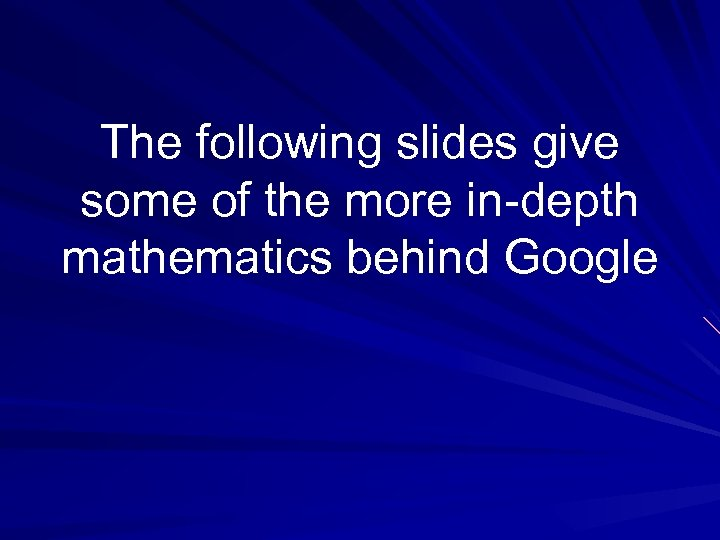The following slides give some of the more in-depth mathematics behind Google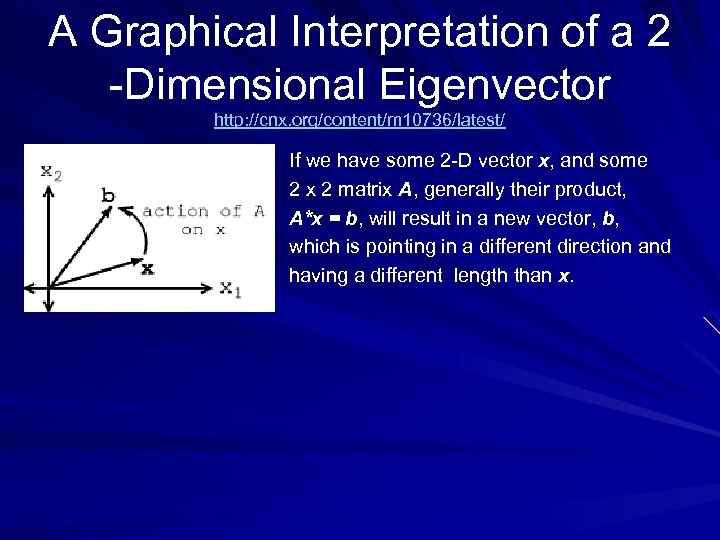A Graphical Interpretation of a 2 -Dimensional Eigenvector http: //cnx. org/content/m 10736/latest/ If we have some 2 -D vector x, and some 2 x 2 matrix A, generally their product, A*x = b, will result in a new vector, b, which is pointing in a different direction and having a different length than x.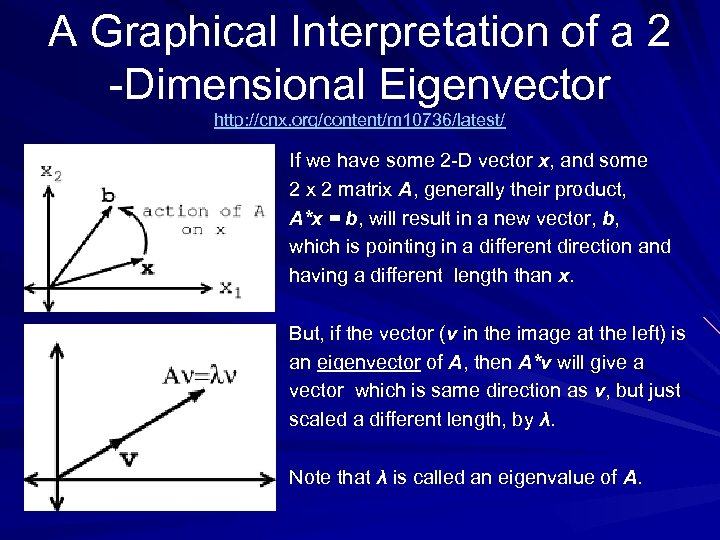A Graphical Interpretation of a 2 -Dimensional Eigenvector http: //cnx. org/content/m 10736/latest/ If we have some 2 -D vector x, and some 2 x 2 matrix A, generally their product, A*x = b, will result in a new vector, b, which is pointing in a different direction and having a different length than x. But, if the vector (v in the image at the left) is an eigenvector of A, then A*v will give a vector which is same direction as v, but just scaled a different length, by λ. Note that λ is called an eigenvalue of A.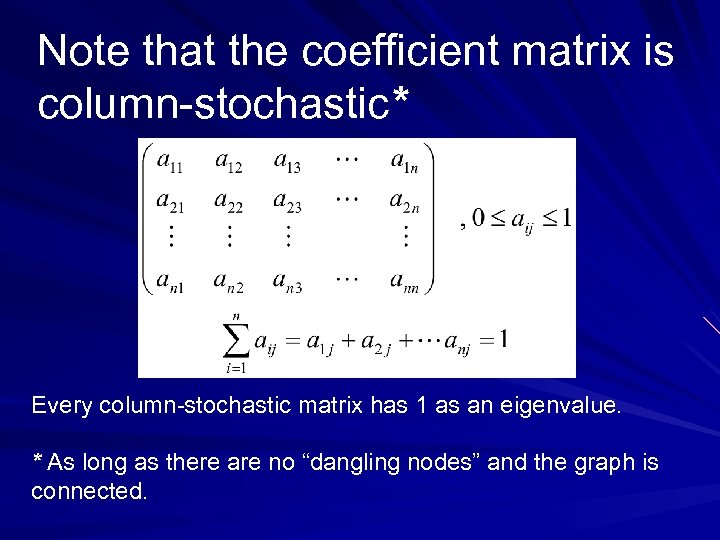Note that the coefficient matrix is column-stochastic* Every column-stochastic matrix has 1 as an eigenvalue. * As long as there are no “dangling nodes” and the graph is connected.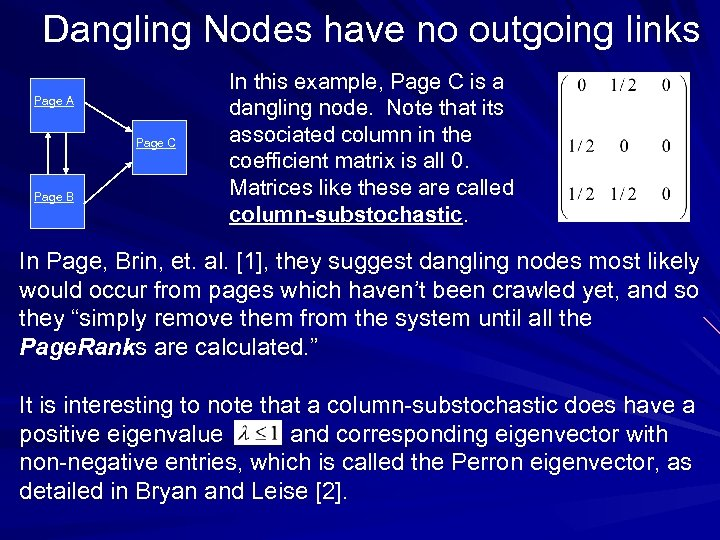Dangling Nodes have no outgoing links Page A Page C Page B In this example, Page C is a dangling node. Note that its associated column in the coefficient matrix is all 0. Matrices like these are called column-substochastic. In Page, Brin, et. al. , they suggest dangling nodes most likely would occur from pages which haven’t been crawled yet, and so they “simply remove them from the system until all the Page. Ranks are calculated. ” It is interesting to note that a column-substochastic does have a positive eigenvalue and corresponding eigenvector with non-negative entries, which is called the Perron eigenvector, as detailed in Bryan and Leise .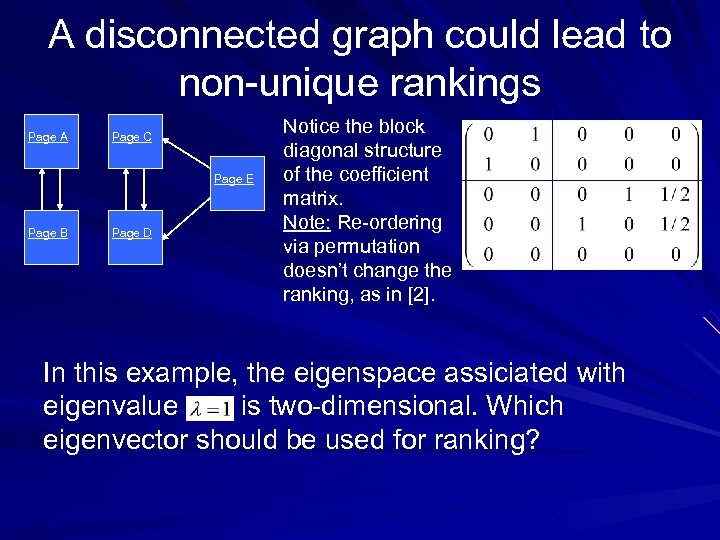A disconnected graph could lead to non-unique rankings Page A Page C Page E Page B Page D Notice the block diagonal structure of the coefficient matrix. Note: Re-ordering via permutation doesn’t change the ranking, as in . In this example, the eigenspace assiciated with eigenvalue is two-dimensional. Which eigenvector should be used for ranking?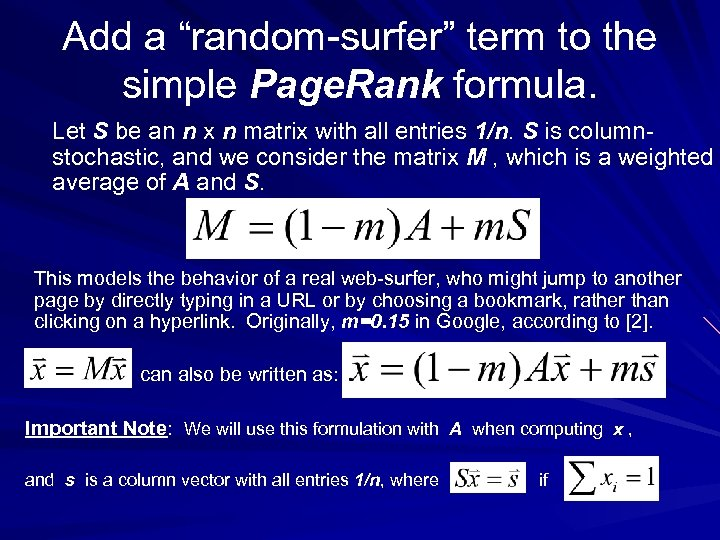Add a “random-surfer” term to the simple Page. Rank formula. Let S be an n x n matrix with all entries 1/n. S is columnstochastic, and we consider the matrix M , which is a weighted average of A and S. This models the behavior of a real web-surfer, who might jump to another page by directly typing in a URL or by choosing a bookmark, rather than clicking on a hyperlink. Originally, m=0. 15 in Google, according to . can also be written as: Important Note: We will use this formulation with A when computing x , and s is a column vector with all entries 1/n, where if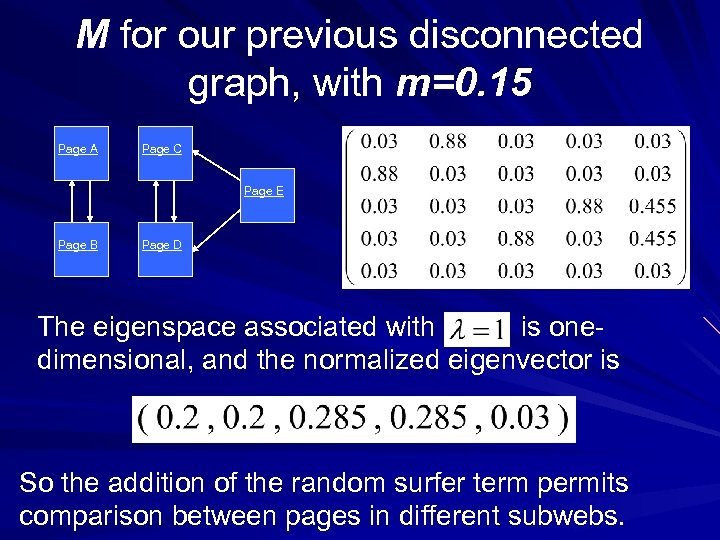M for our previous disconnected graph, with m=0. 15 Page A Page C Page E Page B Page D The eigenspace associated with is onedimensional, and the normalized eigenvector is So the addition of the random surfer term permits comparison between pages in different subwebs.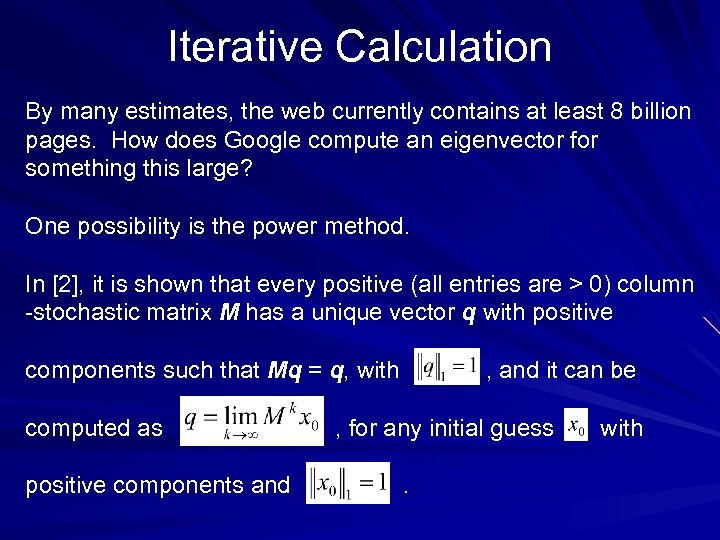Iterative Calculation By many estimates, the web currently contains at least 8 billion pages. How does Google compute an eigenvector for something this large? One possibility is the power method. In , it is shown that every positive (all entries are > 0) column -stochastic matrix M has a unique vector q with positive components such that Mq = q, with computed as positive components and , and it can be , for any initial guess. withIterative Calculation continued Rather than calculating the powers of M directly, we could use the iteration, . Since M is positive, would be an calculation. As we mentioned previously, Google uses the equivalent expression in the computation: These products can be calculated without explicitly creating the huge coefficient matrix, since A contains mostly 0’s. The iteration is guaranteed to converge, and it will converge quicker with a better first guess, so the previous Page. Rank vector is used as the initial vector.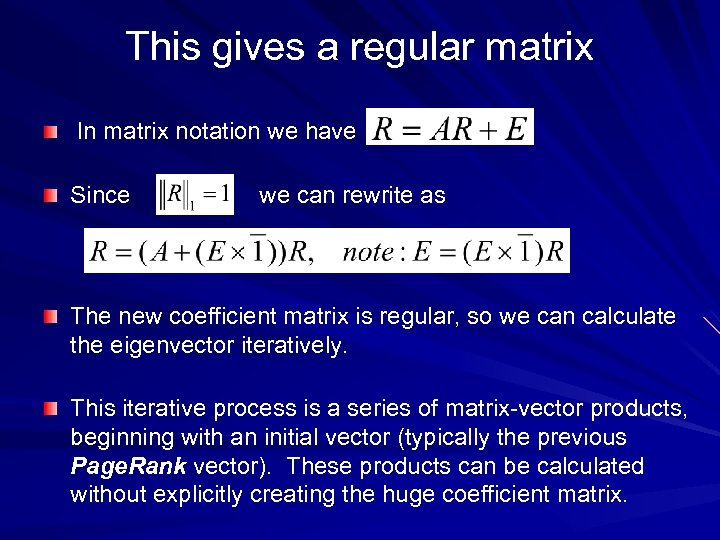This gives a regular matrix In matrix notation we have Since we can rewrite as The new coefficient matrix is regular, so we can calculate the eigenvector iteratively. This iterative process is a series of matrix-vector products, beginning with an initial vector (typically the previous Page. Rank vector). These products can be calculated without explicitly creating the huge coefficient matrix.